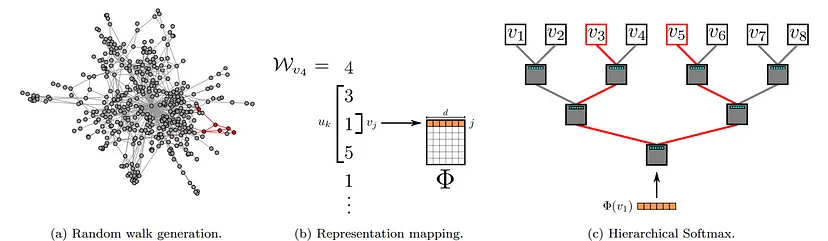LucaSantagata

# Deep Walk

DeepWalk is a type of graph neural network that uses language model to learn latent representations of vertices in a network. DeepWalk includes two main components: Firstly, it uses random walk to infer main local components in the graph. Secondly, it uses SkipGram algorithm to train parameters for embeddings.

### Node embeddings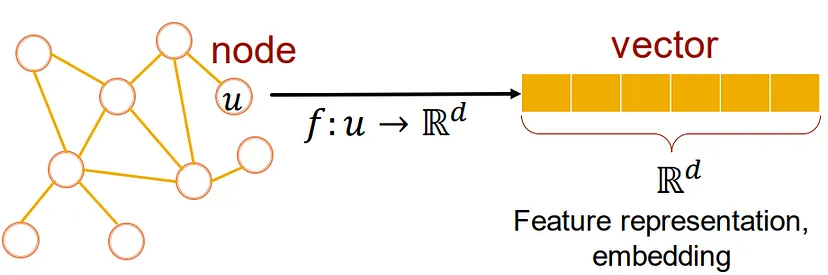The general idea of node embedding is to map nodes into an embedding space that has less dimension than the number of nodes in the graph. In embedding space, it retains some of the same properties as in the graph. The similarity of a pair of nodes in the graph corresponds to their similarity in the embedding space. For example, if two nodes are connected on a graph, they will be close together in the embedding space.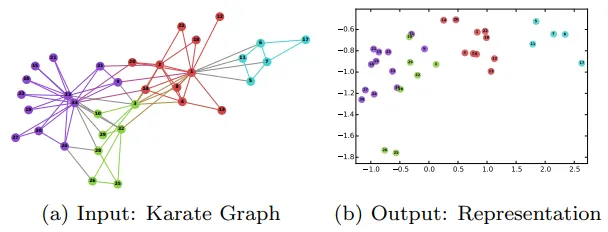### DeepWalk Algorithm

Let G = (V, E) is a graph, where V is the vertices set of the graph and E is the edges set of the graph, and E ⊆ (V × V ).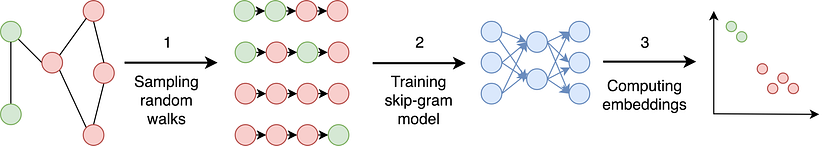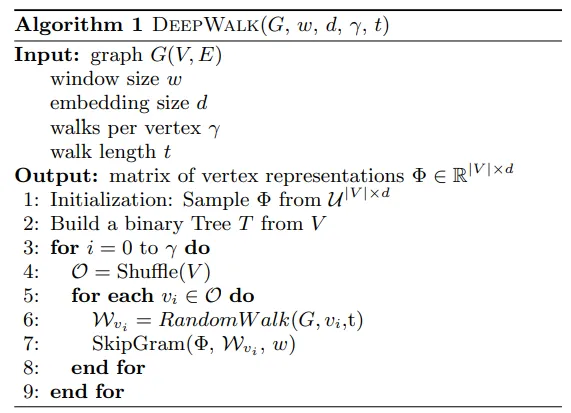The algorithm consists of two main components: first a random walk generator and second an update procedure by SkipGram.

A random walk on a graph is a process that begins at some vertex, and at each time step moves randomly to another vertex. In DeepWalk algorithm, we have $\gamma$ random walks for each vertex. Each random walk has length $t$. Example in figure below, for vertex 0, we generate a random walk with length t = 5. Random walk may be 0 → 1 →2 → 7 → 3 → 1 or 0 → 4 → 6 → 4 → 10 →5,…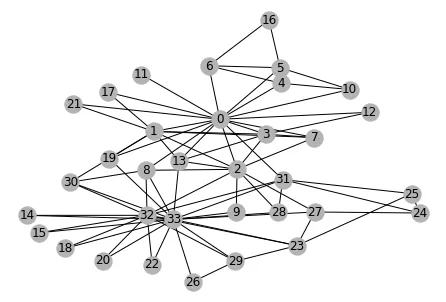Thus, we have sampled random walks from the original graph. After that, the authors use SkipGram for node embedding. SkipGram is a language model that maximizes the cooccurrence probability among the words that appear within a window, w, in a sentence.Thus, we have sampled random walks from the original graph. After that, the authors use SkipGram for node embedding. SkipGram is a language model that maximizes the cooccurrence probability among the words that appear within a window, $w$, in a sentence.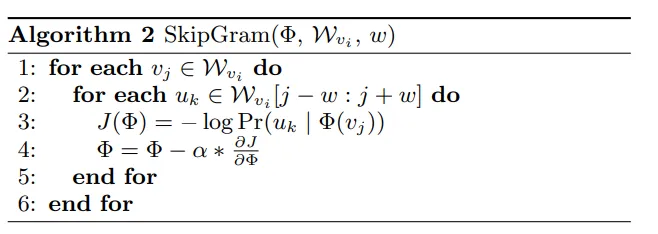For each vertex in walk, the algorithm will maximize the probability of its neighbors in the window size. The model can learn such posterior distribution using several choices of classifiers. However, calculating $Pr(u_k | Φ(vj ))$is not feasible. The authors propose to assign the vertices to the leaves of a binary tree, the prediction problem turns into maximizing the probability of a specific path in the tree.